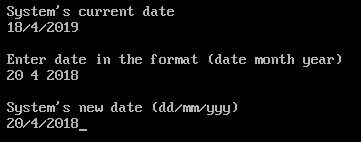Open in App
Not now

# getdate() and setdate() function in C with Examples

• Difficulty Level : Basic
• Last Updated : 13 May, 2022

### setdate() Method:

getdate() function is defined in dos.h header file. This function fills the date structure *dt with the system’s current date. Syntax

```struct date dt;
getdate(&dt);```

Parameter: This function accepts a single parameter dt which is the object of structure date.

Return Value: This method does not return anything. It just gets the system date and sets it in the specified structure.

Note: The <dos.h> header file used in this tutorial works only on dos-based systems and will not work on Linux-based systems.

Program 1: Implementation of getdate() function

## C

 `// C program to demonstrate getdate() method` `#include ``#include ` `int` `main()``{``    ``struct` `date dt;` `    ``// This function is used to get``    ``// system's current date``    ``getdate(&dt);` `    ``printf``("System's current date\n");``    ``printf``("%d/%d/%d",``           ``dt.da_day,``           ``dt.da_mon,``           ``dt.da_year);` `    ``return` `0;``}`

Output:

### setdate() Method:

setdate() function is defined in dos.h header file. This function sets the system date to the date in *dt. Syntax

```struct date dt;
setdate(&dt)```

Parameter: This function accepts a single parameter dt which is the object of structure date that has to be set as the system date. Return Value: This method do not returns anything. It just sets the system date as specified. Program 1: Implementation of setdate() function

## C

 `// C program to demonstrate setdate() method` `#include ``#include ` `int` `main()``{``    ``struct` `date dt;` `    ``// This function is used to get``    ``// system's current date``    ``getdate(&dt);` `    ``printf``("System's current date\n");``    ``printf``("%d/%d/%d",``           ``dt.da_day,``           ``dt.da_mon,``           ``dt.da_year);` `    ``printf``("Enter date in the format (date month year)\n");``    ``scanf``("%d%d%d", &dt.da_day, &dt.da_mon, &dt.da_year);` `    ``// This function is used to change``    ``// system's current date``    ``setdate(&dt);` `    ``printf``("System's ``new` `date (dd/mm/yyyy)\n")``        ``printf``("%d%d%d", dt.da_day, dt.da_mon, dt.da_year);` `    ``return` `0;``}`

Output:Note: These programs are run in TurboC/C++ compiler

My Personal Notes arrow_drop_up